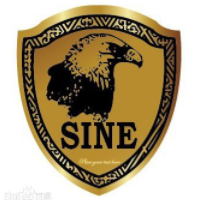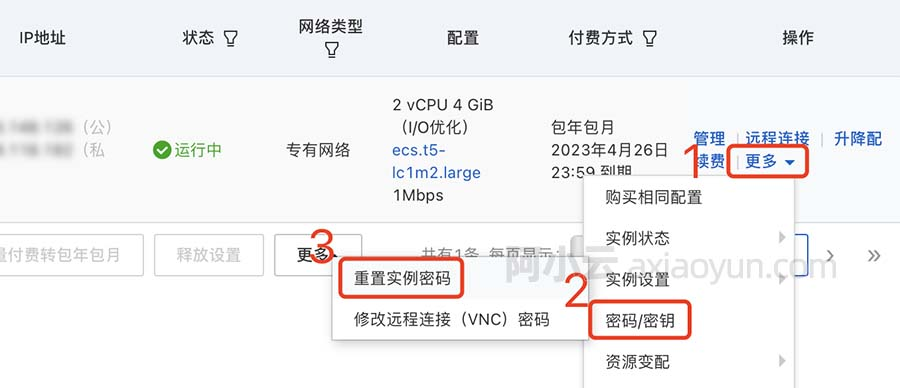# 【代数结构】群 ( 群的定义 | 群的基本性质 | 群的证明方法 | 交换群 )

+关注继续查看

1. 封闭性 :

1> 符号表示 : ∀ a , b ∈ G , a × b = c ∈ G \forall a,b \in G , a \times b = c \in G∀a,b∈G,a×b=c∈G

2> 自然语言描述 : 非空集合 G GG 中任意两个元素 a , b a,ba,b 相乘, 其结果 c cc 也是 集合 G GG 中的元素 ;

2. 结合律 :

3. 有单位元 :

1> 符号表示 : ∃ e ∈ G , ∀ a ∈ G , e × a = a × e = a \exist e \in G, \forall a \in G, e \times a = a\times e = a∃e∈G,∀a∈G,e×a=a×e=a

2> 自然语言描述 : 存在一个 e ee , 乘以 a aa , 或者 与 a aa 相乘 , 其结果都是 a aa , 相当于 1 11 ;

4. 每个元 a aa 有逆元 a − 1 a^{-1}a

−1

:

1> 符号表示 : ∃ e ∈ G , ∀ a ∈ G , ∃ a − 1 ∈ G , a − 1 × a = a × a − 1 = e \exist e \in G, \forall a \in G, \exist a^{-1} \in G, a^{-1} \times a = a \times a^{-1} = e∃e∈G,∀a∈G,∃a

−1

∈G,a

−1

×a=a×a

−1

=e ,

2> 自然语言描述 : e ee 是之前的 单位元 ( 类似于 1 11 ) , a aa 与 a aa 的逆 相乘 , 结果是单位元 e ee ;

G × G G \times GG×G 构成代数结构可以表示成 ( G , ⋅ ) ( G , \cdot )(G,⋅)

1.交换群 ( Abel 群 ) : 交换律 成立的 群 , 称为 交换群 或 Abel 群 ;

2.非交换群 ( 非 Abel 群 ) : 交换律 不成立的 群 , 称为 非交换群 或 非 Abel 群 ;

3.群 的 阶 : 群 G GG 含有的元素个数叫群的阶 , 记做 ∣ G ∣ |G|∣G∣ ;

4.有限群 : ∣ G ∣ |G|∣G∣ 是 有限的 , 叫做 有限群 ;

5.无限群 : ∣ G ∣ |G|∣G∣ 是 无限的 , 叫做 无限群 ;

1.非空集合 : 首先说明 该集合是一个非空集合 ;

2.证明封闭性 : 集合 中 任意两个元素 进行运算 得到的 第三个元素 必须也在 集合中 ;

3.证明结合律 : 集合中 a aa 与 b bb 和 c cc 进行二元运算 , 其结果 与 a aa 和 b bb 与 c cc 进行运算结果相同 ;

4.证明其有单位元 : 集合中存在一个 e ee 元素 , a aa 与 e ee 和 e ee 与 a aa 运算 结果都是 a aa ; 相当于乘法中的 1 11 或 加法中的 0 00 ;

5.证明其逆元 : a aa 与 a − 1 a^{-1}a

−1

或者 a − 1 a^{-1}a

−1

与 a aa 进行运算 , 其结果是 e ee 单位元 ;

1.整数 : Z ZZ , 所有整数组成的集合 , 称为 整数集 ;

2.正整数 : Z + , N ∗ , N + Z^+,N^*,N^+Z

+

,N

,N

+

, 所有正整数组成的集合 , 称为正整数集 ;

3.负整数 : Z − Z^-Z

, 所有负整数组成的集合 , 称为负整数集 ;

4.非负整数 : N NN , 所有非负整数组成的集合 , 称为非负整数集 ( 或 自然数集 ) ;

5.有理数 : Q QQ , 全体有理数 组成的集合 , 称为有理数集 ;

6.实数集 : R RR , 全体实数组成的集合 , 称为实数集 ;

7.虚数 : I II , 全体虚数组成的集合 , 称为虚数集 ;

8.复数 : C CC , 全体实数 和 虚数 组成的集合 , 称为复数集 ;

1.正数 : + ^+

+

表示该数集中元素全为 正数 ;

2.负数 : − ^-

表示该数集中的元素全为 负数 ;

3.剔除 0 00 元素 : ∗ ^*

表示剔除该数集上的元素 0 00 ;

R ∗ R^*R

表示剔除 实数集 R RR 中的 元素 0 00 ,

R ∗ = R ∖ { 0 } = R − ∪ R + = ( − ∞ , 0 ) ∪ ( 0 , + ∞ ) R^* = R \setminus \{0\} = R^- \cup R^+ = (- \infty , 0) \cup (0,+ \infty)R

=R∖{0}=R

∪R

+

=(−∞,0)∪(0,+∞)

1.非空集合 : 首先说明 该集合是一个非空集合 ;

2.证明封闭性 : 集合 中 任意两个元素 进行运算 得到的 第三个元素 必须也在 集合中 ;

3.证明结合律 : 集合中 a aa 与 b bb 和 c cc 进行二元运算 , 其结果 与 a aa 和 b bb 与 c cc 进行运算结果相同 ;

4.证明其有单位元 : 集合中存在一个 e ee 元素 , a aa 与 e ee 和 e ee 与 a aa 运算 结果都是 a aa ; 相当于乘法中的 1 11 或 加法中的 0 00 ;

5.证明其逆元 : a aa 与 a − 1 a^{-1}a

−1

或者 a − 1 a^{-1}a

−1

与 a aa 进行运算 , 其结果是 e ee 单位元 ;

① 封闭性 : 有理数 相乘 肯定也是有理数 , 满足封闭性 ;

② 结合律 : 3 33 个 任意 有理数 相乘 , 显然也是 满足 结合律的 ;

③ 证明单位元 : 存在 e = 1 e=1e=1 , 有理数 乘以 1 或者 1 乘以 有理数 , 都等于该有理数 , 说明单位元存在 ;

④ 证明逆 a − 1 a^{-1}a

−1

的存在 : 集合中的任意元素 a aa , 其 a − 1 = 1 a a^{-1} = \frac{1}{a}a

−1

=

a

1

, a − 1 × a = a × a − 1 = e = 1 a^{-1} \times a = a \times a^{-1} = e = 1a

−1

×a=a×a

−1

=e=1 , 其逆元成立 ;12123 022717 08734 0windows server 2008阿里云ECS服务器安全设置

11658 016922 019394 034369 021213 059096 024449 0

2605

0

OceanBase 入门到实战教程